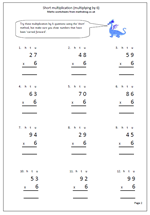# Short multiplication by 6Here is the latest worksheet which looks at the short multiplication method; this time multiplying 2-digit numbers by 6. It is called the short method because it eliminates the process of putting parts of the answer in two or three columns below, as in the ‘long’ method. The stages are the same as before:

1. writing the question out correctly so that it is in vertical format. it is a good idea to write a small h, t and u above to show the hundreds, tens and units columns.

2. Multiplying the units and carrying any of the tens across, below the answer line.

3. multiplying the tens and putting any hundreds into the answer.

More clarification of this method is shown on the worksheet.

The 6 times table is one of the harder tables to learn, but knowing it off by heart makes this type of multiplication so much easier.

Short multiplication by 6